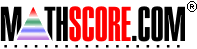Math Practice Online > free > lessons > Florida > 6th grade > Function Tables 2

## Function Tables 2

Function tables are also input/output tables. In this topic, the student will
• Fill out missing values in the table
• Apply the rule to the table
• Find the rule for the table
• Interpret word problems into algebraic rules

 Sample Problems for Function Tables 2 Lesson for Function Tables 2

### This topic aligns to the following state standards

Grade 1: Alg 2. Describes a pattern rule.
Grade 2: Alg 2. Describes a given pattern and explains the pattern rule.
Grade 5: Alg 3. Explains and expresses numerical relationships and pattern generalizations, using algebraic symbols (for example, in the problem above, the number of calls on the nth day can be expressed as 3n+1).
Grade 3: Alg 2. Analyzes number patterns and states the rule for relationships (for example, 2, 4, 6, 8, ... the rule: +2).
Grade 3: Alg 4. Identifies and extends a pattern according to the given rule.
Grade 4: Alg 2. Analyzes number patterns and states rules for relationships (for example, 2, 4, 7, 9, 12, ... the rule is: +2, +3, +2, +3, ...).
Grade 5: Alg 2. Analyzes and generalizes number patterns and states the rule for relationships (for example, 1, 4, 9, 16, ... the rule: +3, +5, +7, ... or "squares of the whole numbers").
Grade 6: Alg 5. Describes relationships and patterns using words, tables, symbols, variables, expressions, or equations.
Grade 7: Alg 2. Given instances of a pattern, expresses a generalization of the pattern using algebraic expressions.
Grade 8: Alg 4. Finds a function rule to describe tables of related input-output variables.
Grade 9: Alg 1. describes, analyzes, and generalizes relationships, patterns, and functions using words, symbols, variables, tables, and graphs
Grade 1: Alg 5. Identifies and generates patterns in a list of related number pairs based on real-life situations (for example, T-chart with number of children to number of eyes).
Grade 2: Alg 6. Identifies and generates patterns in a list of related number pairs based on real-life situations (for example, T-chart with number of tricycles to number of wheels).
Grade 3: Alg 5. Applies and explains the appropriate rule to complete a table or chart (for example, in the following table, the rule is "multiply by 6"): 1 2 3 4   6 12 ? 24
Grade 4: Alg 4. Applies the appropriate rule to complete a table or a chart such as: Input 2 9 ? 7   Output 8 36 16 28
Grade 5: Alg 3. Applies the appropriate rule to complete a table or a chart, such as: IN 1 2 3 9   OUT 1 4 9 ?
Grade 6: Alg 1. Describes, predicts, and creates numerical and geometric patterns through models (for example, manipulatives, tables, graphs).

Copyright Accurate Learning Systems Corporation 2008.